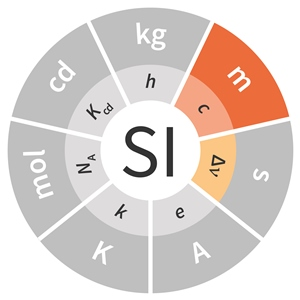# SI base unit: metre (m)

The metre, symbol m, is the SI unit of length. It is defined by taking the fixed numerical value of the speed of light in vacuum c to be 299 792 458 when expressed in the unit m s–1, where the second is defined in terms of the caesium frequency ΔνCs.This definition implies the exact relation c = 299 792 458 m s–1. Inverting this relation gives an exact expression for the metre in terms of the defining constants c and ΔνCs:The effect of this definition is that one metre is the length of the path travelled by light in vacuum during a time interval with duration of 1/299 792 458 of a second.